# Joint-Space Motion Model

The joint-space motion model characterizes the closed-loop motion of a manipulator under joint-space control, where the control action is defined in the joint configuration space. Motion models are used as low-fidelity plant models of robots under closed-loop position control. This topic covers the variables and equations for computing the behavior of the joint-space position, velocity, and accelerations relative to reference inputs, as used in the `jointSpaceMotionModel` object. For task-space motion models, see the Plan and Execute Task- and Joint-Space Trajectories Using KINOVA Gen3 Manipulator object.

This topic covers these types of joint-space control: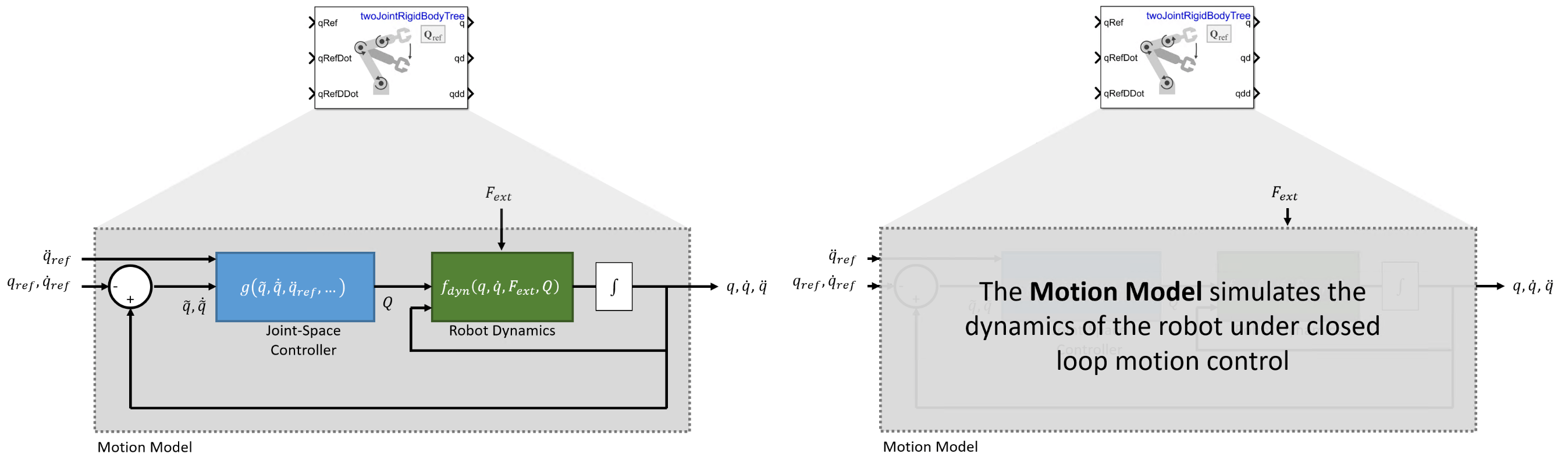For an example that covers the difference between task-space and joint-space control, see Plan and Execute Task- and Joint-Space Trajectories Using KINOVA Gen3 Manipulator.

### State and Controls

The joint-space motion model state consists of these values:

• $q$ — Robot joint configuration, as a vector of joint positions. Specified in $\mathrm{rad}$ for revolute joints and $\mathit{m}$ for prismatic joints.

• $\underset{}{\overset{˙}{q}}$ — Vector of joint velocities in $rad\cdot {s}^{-1}$ for revolute joints and $m\cdot {s}^{-1}$ for prismatic joints

• $\underset{}{\overset{¨}{q}}$ — Vector of joint accelerations in $rad\cdot {s}^{-2}$ for revolute joints or $m\cdot {s}^{-2}$ for prismatic joints

The joint-space motion model is used when you need a low-fidelity model of your system under closed-loop control and the inputs are specified as joint configuration, velocity, and acceleration. The motion model includes three ways to model its overall behavior:

• Computed Torque Control — The rigid-body dynamics are modeled using the standard equations of motion, but compensating for the full-body dynamics and assigning error dynamics. This is a higher-fidelity version of independent joint motion control.

• PD Control — The rigid-body dynamics are modeled using the standard equations of motion with a joint torque input given by proportional-derivative (PD) control. This model represents a controller that does not compensate tightly for the overall effects of rigid-body motion.

• Independent Joint Motion — Each joint is modeled independently as a closed-loop second-order system. This model is a lower fidelity version of computed torque control motion model, and may be considered a best-case scenario for how closed-loop motion may behave since the dynamics are simplified and directly prescribed.

To set these different motion types, use the MotionType property of the `jointSpaceMotionModel` object. These motion types are not exhaustive, but they do provide a set of options to use when approximating the closed-loop behavior of your system. For details and suggestions on when to use which model, see the sections below.

### Equations of Motion

In this section, the equations of motion for each model are introduced, in order of decreasing complexity.

#### Computed Torque Control

With computed torque control, the motion model uses standard rigid body dynamics, but the generalized force input is given by a control law that compensates for the rigid body dynamics and instead assigns a second-order error dynamics response.

• Inputs — This model accepts ${q}_{ref},{\underset{}{\overset{˙}{q}}}_{ref},{\underset{}{\overset{¨}{q}}}_{ref}$ as the desired reference joint configuration, velocities, and accelerations as vectors. The user may also optional provide the external force ${F}_{ext}$, specified in Newtons.

• Outputs — The model outputs $q,\underset{}{\overset{˙}{q}},\underset{}{\overset{¨}{q}}$ as the joint configuration, velocities, and accelerations as vectors. In the MATLAB version of the model, only accelerations are returned, and the user must choose an integrator or ODE solver to return the other states.

• Complexity — This is high complexity. The motion model uses full rigid body dynamics with optional external forces, the controller is modeled as part of the closed loop system, and the controller includes dynamic compensation terms.

• When to apply — Use when the closed-loop system being simulated has approximable error dynamics, or when it uses a controller that treats the robot as a multi-body system, and external forces may be present

The resultant closed-loop system aims to achieve the following second error behavior for the $\mathit{i}$-th joint:

`${\underset{}{\overset{¨}{\underset{}{\overset{\sim }{q}}}}}_{i}=-{\omega }_{n}^{2}{\underset{}{\overset{\sim }{q}}}_{i}-2\zeta {\omega }_{n}{\underset{}{\overset{˙}{\underset{}{\overset{\sim }{q}}}}}_{i}$`

`${\underset{}{\overset{\sim }{q}}}_{i}={q}_{i}-{q}_{i.ref}$`

These parameters characterize the desired response defined for each joint:

• ${\omega }_{n}$ — Natural frequency, specified in Hz (${s}^{-1}$)

• $\zeta$ — The damping ratio, which is unitless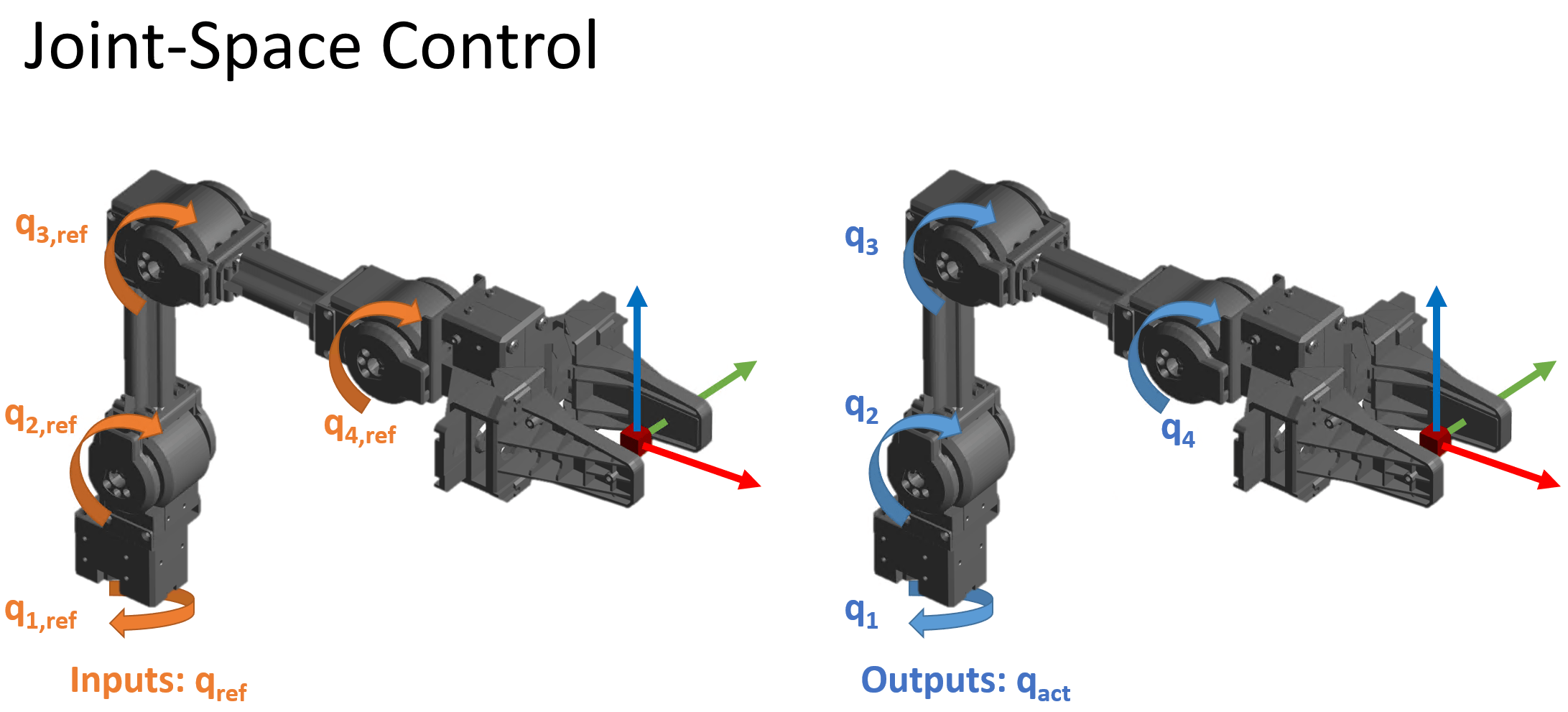As seen in the diagram, the complete system consists of the standard rigid-body Robot Dynamics with a control law that enforces closed error dynamics via the generalized force input $Q$:

`$\frac{d}{dt}\left[\begin{array}{c}q\\ \underset{}{\overset{˙}{q}}\end{array}\right]={f}_{dyn}\left(q,\underset{}{\overset{˙}{q}},Q,{F}_{ext}\right)$`

`$Q={g}_{CTC}\left(\underset{}{\overset{\sim }{q}},\underset{}{\overset{˙}{\underset{}{\overset{\sim }{q}}}},{\underset{}{\overset{¨}{q}}}_{ref},{\omega }_{n},\zeta \right)=M\left(q\right){a}_{q}+C\left(q,\underset{}{\overset{˙}{q}}\right)\underset{}{\overset{˙}{q}}+G\left(q\right)$`

`${a}_{q}={\underset{}{\overset{¨}{q}}}_{ref}-{\left[{\omega }_{n}^{2}\right]}_{diag}\underset{}{\overset{\sim }{q}}-{\left[2\zeta {\omega }_{n}\right]}_{diag}\underset{}{\overset{˙}{\underset{}{\overset{\sim }{q}}}}$`

`$\underset{}{\overset{\sim }{q}}=q-{q}_{ref}$`

where,

• $M\left(q\right)$ — is a joint-space mass matrix based on the current robot configuration Calculate this matrix by using the `massMatrix` object function.

• $C\left(q,\underset{}{\overset{˙}{q}}\right)$ — is the coriolis terms, which are multiplied by $\underset{}{\overset{˙}{q}}$ to calculate the velocity product. Calculate the velocity product by using by the `velocityProduct` object function.

• $G\left(q\right)$ — is the gravity torques and forces required for all joints to maintain their positions in the specified gravity Gravity. Calculate the gravity torque by using the `gravityTorque` object function.

The control input relies on these user-defined parameters:

• ${\left[-{\omega }_{n}^{2}\right]}_{diag}$ — Diagonal matrix, where the $\left(\mathit{i},\mathit{i}\right)$-th element corresponds to the $\mathit{i}$-th element of the n-element vector of natural frequencies in the NaturalFrequency property of the `jointSpaceMotionModel` object are in Hz (${s}^{-1}$).

• ${\left[-2\zeta {\omega }_{n}^{2}\right]}_{diag}$ — Diagonal matrix, where the $\left(\mathit{i},\mathit{i}\right)$-th element corresponds to the $\mathit{i}$-th element of the product of the squared natural frequencies vector ${\omega }_{n}$ and the $\mathit{i}$-th element of the damping ratios vector $\zeta$, specified in the DampingRatio property of the `jointSpaceMotionModel` object.

Because the dynamics are compensated, in the absence of external force inputs large acceleration/deceleration, the error dynamics should be achieved. In the absence of external forces, the independent joint motion type provides a simpler way of achieving this result.

The values of ${\omega }_{n}$ and $\zeta$ may be set directly, or they may be provided using the the `updateErrorDynamicsFromStep` method, which computes values for ${\omega }_{n}$ and $\zeta$ based on desired unit step response (defined using it's transient behavior characteristics).

#### Proportional-Derivative (PD) Control

With PD control, the robot models behavior according to standard rigid body dynamics, but with the generalized force input $Q$ given by a control law that applies PD control based on the joint error, as well as gravity compensation.

• Inputs — This model accepts ${q}_{ref},{\underset{}{\overset{˙}{q}}}_{ref}$ as the desired reference joint configuration, velocities, and accelerations as vectors. The user may also optional provide the external force ${F}_{ext}$, specified in Newtons.

• Outputs — The model outputs $q,\underset{}{\overset{˙}{q}},\underset{}{\overset{¨}{q}}$ as the joint configuration, velocities, and accelerations. In the MATLAB version of the model, only accelerations are returned, and the user must choose an integrator or ODE solver to return the other states.

• Complexity — This is medium complexity. The motion model uses full rigid body dynamics with optional external forces and the controller is modeled as part of the closed loop system, but the controller is relatively simple.

• When to apply — Use when the closed-loop system being simulated uses a controller that treats joints as independent systems, or when a PD style controller is used, and external forces may be present.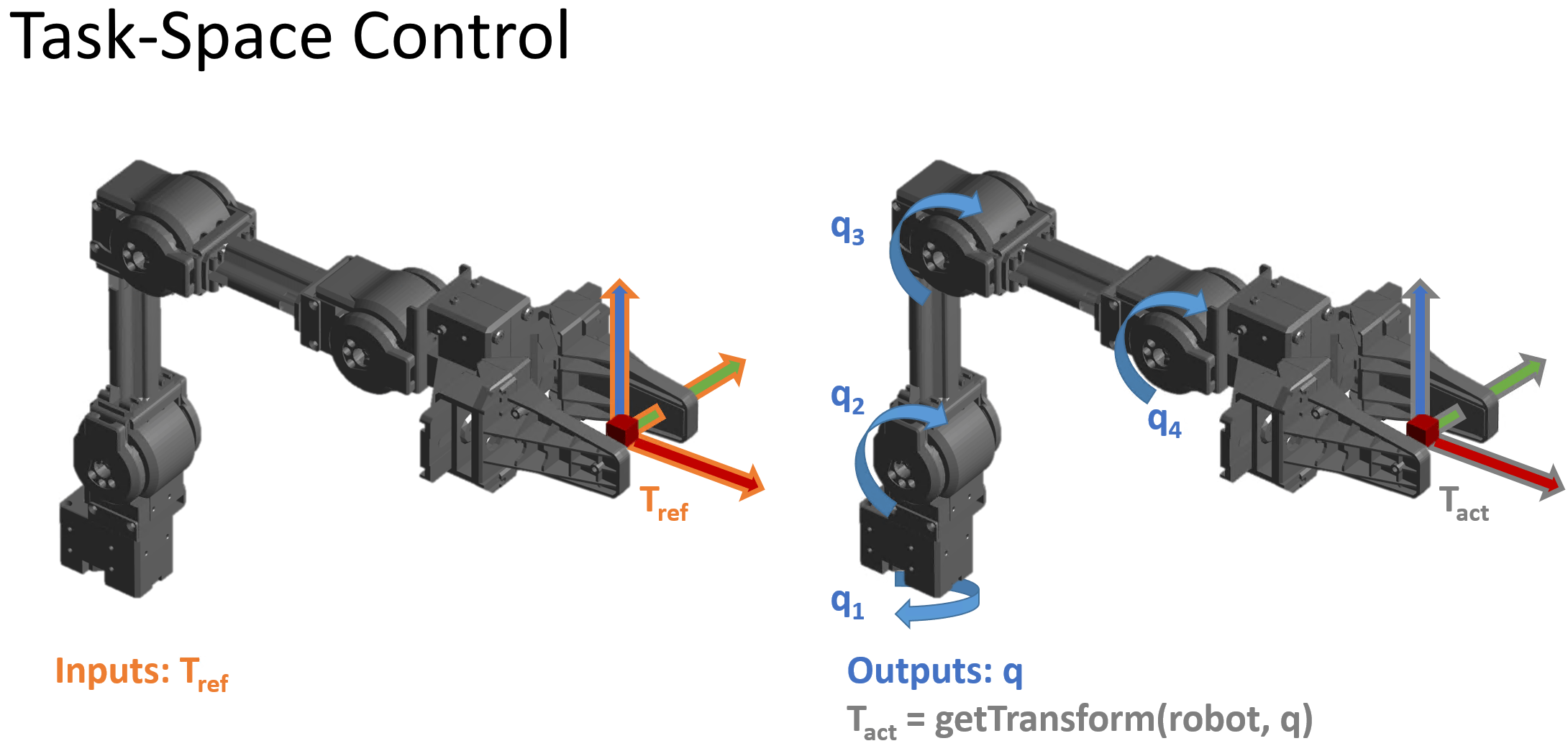As with computed torque control, this system behavior uses the standard rigid-body Robot Dynamics, but uses the PD control law define the generalized force input $Q$:

`$\frac{d}{dt}\left[\begin{array}{c}q\\ \underset{}{\overset{˙}{q}}\end{array}\right]={f}_{dyn}\left(q,\underset{}{\overset{˙}{q}},\tau ,{F}_{ext}\right)$`

`$Q={g}_{PD}\left(\underset{}{\overset{\sim }{q}},\underset{}{\overset{˙}{\underset{}{\overset{\sim }{q}}}},{K}_{P},{K}_{D}\right)=-{K}_{P}\left(\underset{}{\overset{\sim }{q}}\right)-{K}_{D}\left(\underset{}{\overset{˙}{\underset{}{\overset{\sim }{q}}}}\right)+G\left(q\right)$`

`$\underset{}{\overset{\sim }{q}}=q-{q}_{ref}$`

where

• $G\left(q\right)$ — is the gravity torques and forces required for all joints to maintain their positions in the specified gravity Gravity. Calculate the gravity torque by using the `gravityTorque` object function.

The control input relies on these user-defined parameters:

• ${K}_{P}$ — Proportional gain, specified as an $\mathit{N}$-by-$\mathit{N}$ matrix, where $\mathit{N}$ is the number of movable joints of the manipulator

• ${K}_{D}$ — Derivative gain, specified as an $\mathit{N}$-by-$\mathit{N}$ matrix

#### Independent Joint Motion

When this system is modeled with independent joint motion, instead of modeling the closed loop system as standard rigid body dynamics plus a control input, each joint is instead modeled as a second-order system that already has the desired error behavior:

• Inputs — This model accepts ${q}_{ref},{\underset{}{\overset{˙}{q}}}_{ref}$ as the desired reference joint configuration, velocities, and accelerations as vectors. There is no external force input.

• Outputs — The model outputs $q,\underset{}{\overset{˙}{q}},\underset{}{\overset{¨}{q}}$ as the joint configuration, velocities, and accelerations. In the MATLAB version of the model, only accelerations are returned, and the user must choose an integrator or ODE solver to return the other states.

• Complexity — This is low complexity. The motion model simply prescribes the error behavior that a position controller could aim to achieve.

• When to apply — Use when the system has approximable error dynamics and there are no external force inputs required.

The system models the following closed-loop second order behavior for the ith joint:

`$\frac{d}{dt}\left[\begin{array}{c}\underset{}{\overset{\sim }{q}}\\ \underset{}{\overset{˙}{\underset{}{\overset{\sim }{q}}}}\end{array}\right]={f}_{err}\left(\underset{}{\overset{\sim }{q}},\underset{}{\overset{˙}{\underset{}{\overset{\sim }{q}}}},\zeta ,{\omega }_{n}\right)=\left[\begin{array}{c}\underset{}{\overset{˙}{\underset{}{\overset{\sim }{q}}}}\\ -{\omega }_{n}^{2}{\underset{}{\overset{\sim }{q}}}_{i}-2\zeta {\omega }_{n}{\underset{}{\overset{˙}{\underset{}{\overset{\sim }{q}}}}}_{i}\end{array}\right]$`

`${\underset{}{\overset{\sim }{q}}}_{i}={q}_{i}-{q}_{i.ref}$`

These parameters characterize the desired response defined for each joint:

• ${\omega }_{n}$ — the natural frequency specified in units of ${s}^{-1}$

• $\zeta$ — t the damping ratio, which is unitless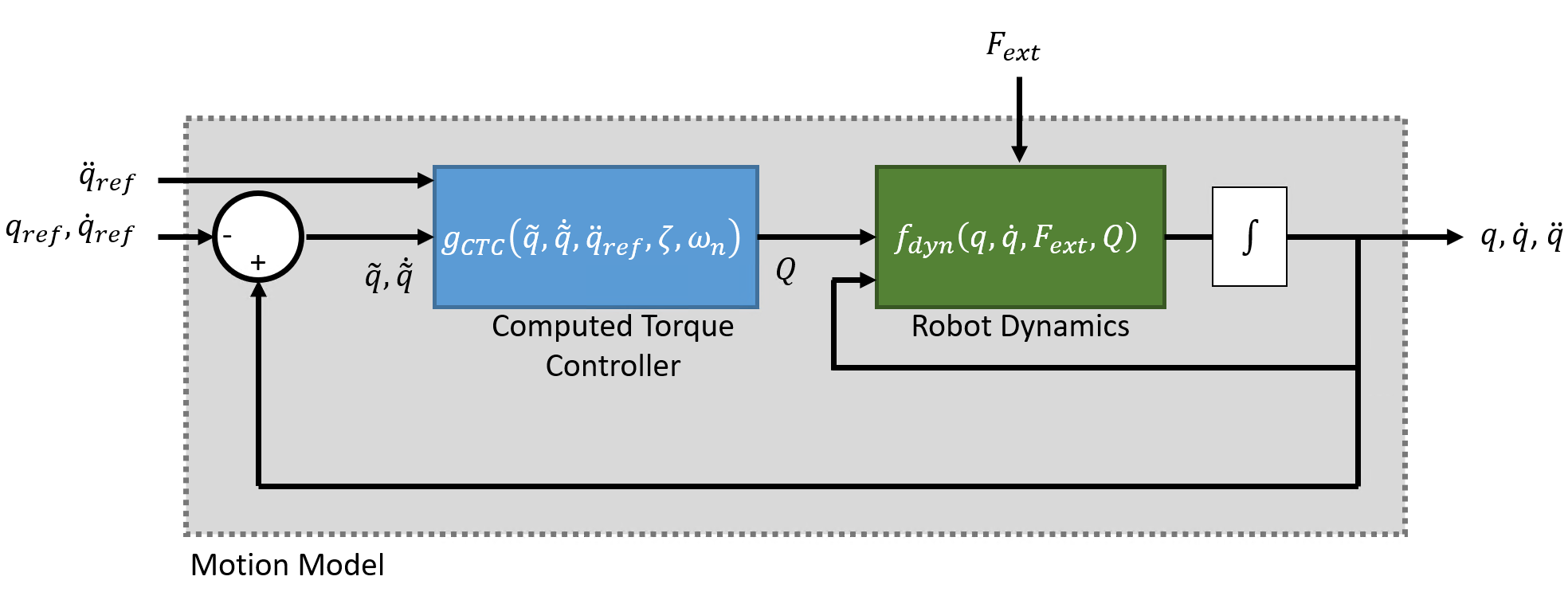Or as: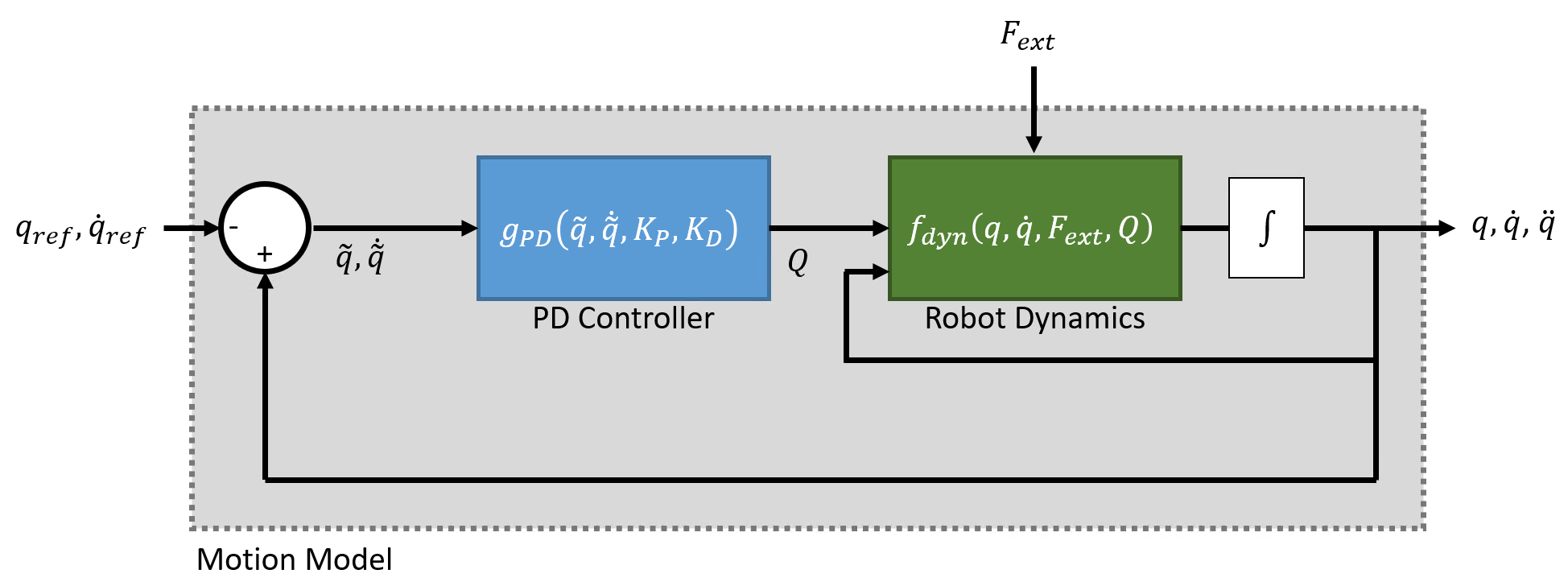The complete system is therefore modeled as:

`$\frac{d}{dt}\left[\begin{array}{c}q\\ \underset{}{\overset{˙}{q}}\end{array}\right]={f}_{IJM}\left({q}_{ref},{\underset{}{\overset{˙}{q}}}_{ref},\zeta ,{\omega }_{n}\right)=\left[\begin{array}{cc}0& I\\ {\left[-{\omega }_{n}^{2}\right]}_{diag}& {\left[-2\zeta {\omega }_{n}\right]}_{diag}\end{array}\right]\left[\begin{array}{c}q\\ \underset{}{\overset{˙}{q}}\end{array}\right]+\left[\begin{array}{cc}0& I\\ {\left[{\omega }_{n}^{2}\right]}_{diag}& {\left[2\zeta {\omega }_{n}\right]}_{diag}\end{array}\right]\left[\begin{array}{c}{q}_{ref}\\ {\underset{}{\overset{˙}{q}}}_{ref}\end{array}\right]$`

The model relies on these user-defined parameters:

• ${\left[-{\omega }_{n}^{2}\right]}_{diag}$ — Diagonal matrix, where the $\left(\mathit{i},\mathit{i}\right)$-th element corresponds to the $\mathit{i}$-th element of the n-element vector of natural frequencies in the NaturalFrequency property of the `jointSpaceMotionModel` object are in Hz (${s}^{-1}$).

• ${\left[-2\zeta {\omega }_{n}^{2}\right]}_{diag}$ — Diagonal matrix, where the $\left(\mathit{i},\mathit{i}\right)$-th element corresponds to the $\mathit{i}$-th element of the product of the squared natural frequencies vector ${\omega }_{n}$ and the $\mathit{i}$-th element of the damping ratios vector $\zeta$, specified in the DampingRatio property of the `jointSpaceMotionModel` object.

The values of ${\omega }_{n}$ and $\zeta$ may be set directly, or they may be provided using the the `updateErrorDynamicsFromStep` method, which computes values for ${\omega }_{n}$ and $\zeta$ based on desired unit step response (defined using it's transient behavior characteristics).

The Independent Joint Motion model represents a closed loop system under idealized behavior. In the absence of external forces, the motion model using computed torque control should produce an equivalent output.